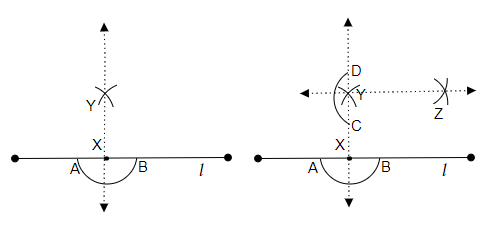# Draw a line $l$ and a point $\mathrm{X}$ on it. Through $\mathrm{X}$, draw a line segment $\overline{\mathrm{XY}}$ perpendicular to $1$. Now draw a perpendicular to $\overline{X Y}$ at Y. (use ruler and compasses)

To do:

We have to draw a perpendicular to $\overline{XY}$ at Y.

Solution:Steps of construction:

(i) Let us draw a line 'l' and point a point X on it.

(ii) Now, let us draw an arc intersecting the line 'l' at two points by placing the pointer of the compasses on point X as the centre and name the intersects of the arc as A and B respectively.

(iii) Then, Let us take the compasses and measure a radius greater than the length from A to X.

(iv) Now, by placing the pointer of the compasses on point X and Point Y respectively let us draw two arcs intersecting each other at point Y.

(v) Now, let's join point X and point Y. Therefore, a line perpendicular to the line 'l' is formed.

(vi) In a similar way, let us draw an arc intersecting the perpendicular line at two points, by placing the pointer of the compasses on point Y as the centre and name the intersects of the arc as C and D respectively.

(vii) Then  Let us take the compasses and measure a radius greater than the length from C to D and by placing the pointer of the compasses on point C and Point D respectively let us draw two arcs intersecting each other at point Z.

(viii) Now, let's join point Y and point Z. Therefore, the line perpendicular to $\overline{XY}$ at Y is formed.

Updated on: 10-Oct-2022

29 Views